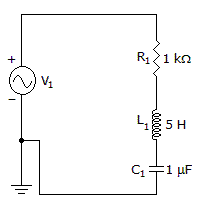# Electronics - RLC Circuits and Resonance

### Exercise :: RLC Circuits and Resonance - General Questions

21.

What is the true power consumed in a 30 V series RLC circuit if Z = 20 ohms and R = 10 ohms?

 A. 15.0 watts B. 22.5 watts C. 30.0 watts D. 45.0 watts

Answer: Option B

Explanation:

No answer description available for this question. Let us discuss.

22.

What is the current phase angle for a parallel RLC circuit when IL = 15.3 A, IC = 0.43 A, and IR = 3.5 A?

 A. 76.7 degrees B. –4.25 degrees C. 88.8 degrees D. –76.7 degrees

Answer: Option D

Explanation:

No answer description available for this question. Let us discuss.

23.

At any resonant frequency, what voltage is measured across the two series reactive components?

 A. applied B. reactive C. zero D. inductive and capacitive

Answer: Option C

Explanation:

No answer description available for this question. Let us discuss.

24.

What is the resonance frequency?A. 31.8 Hz B. 71 Hz C. 7.1 kHz D. 31.8 kHz

Answer: Option B

Explanation:

No answer description available for this question. Let us discuss.

25.

What is the range between f1 and f2 of an RLC circuit that resonates at 150 kHz and has a Q of 30?

 A. 100.0 kHz to 155.0 kHz B. 147.5 kHz to 152.5 kHz C. 4500 kHz to 295.5 kHz D. 149,970 Hz to 150,030 Hz

Answer: Option B

Explanation:

No answer description available for this question. Let us discuss.

#### Current Affairs 2021

Interview Questions and Answers